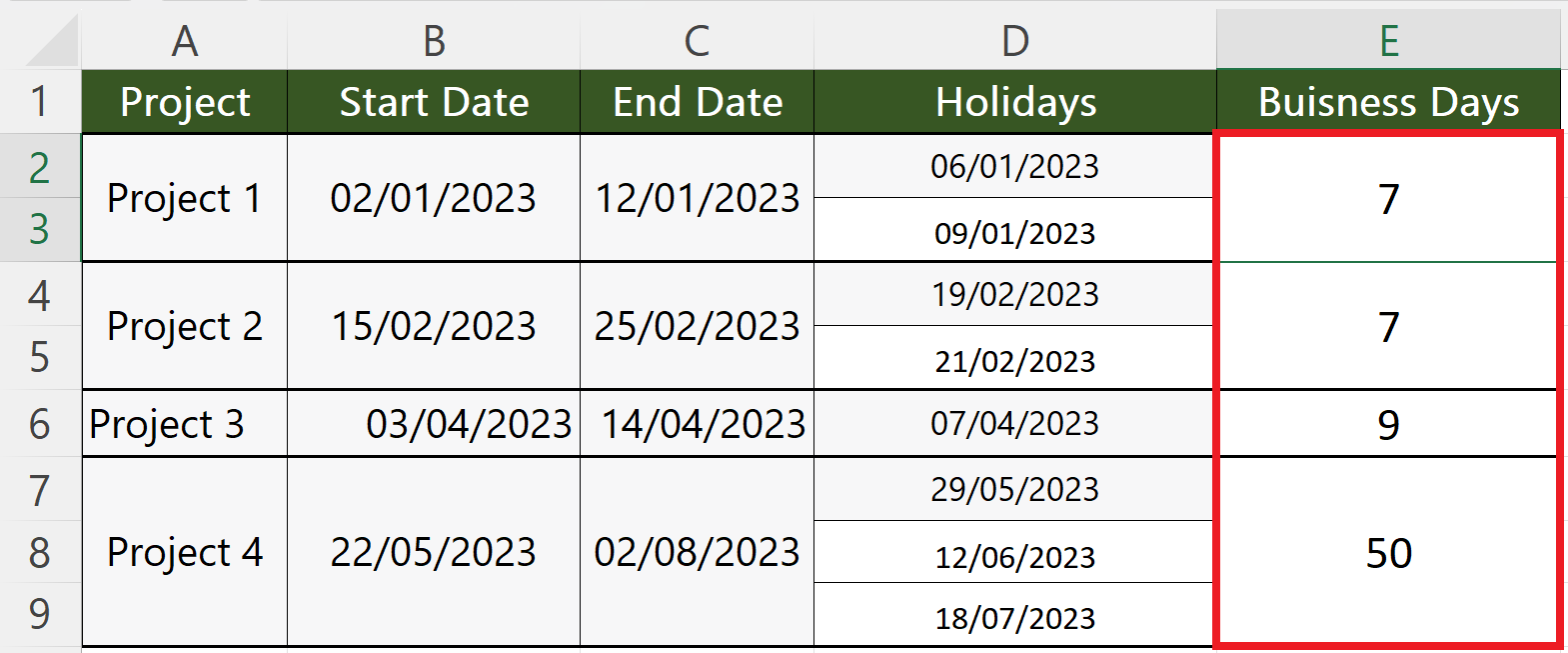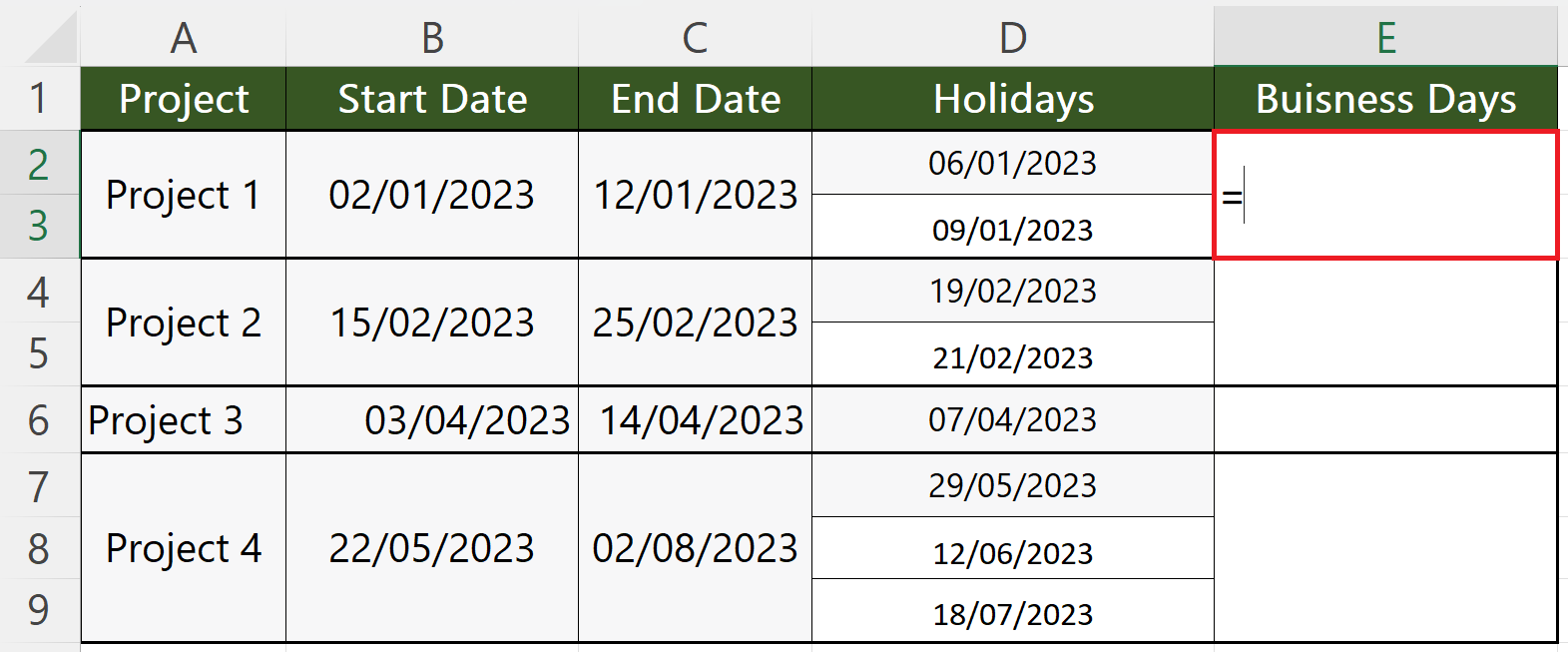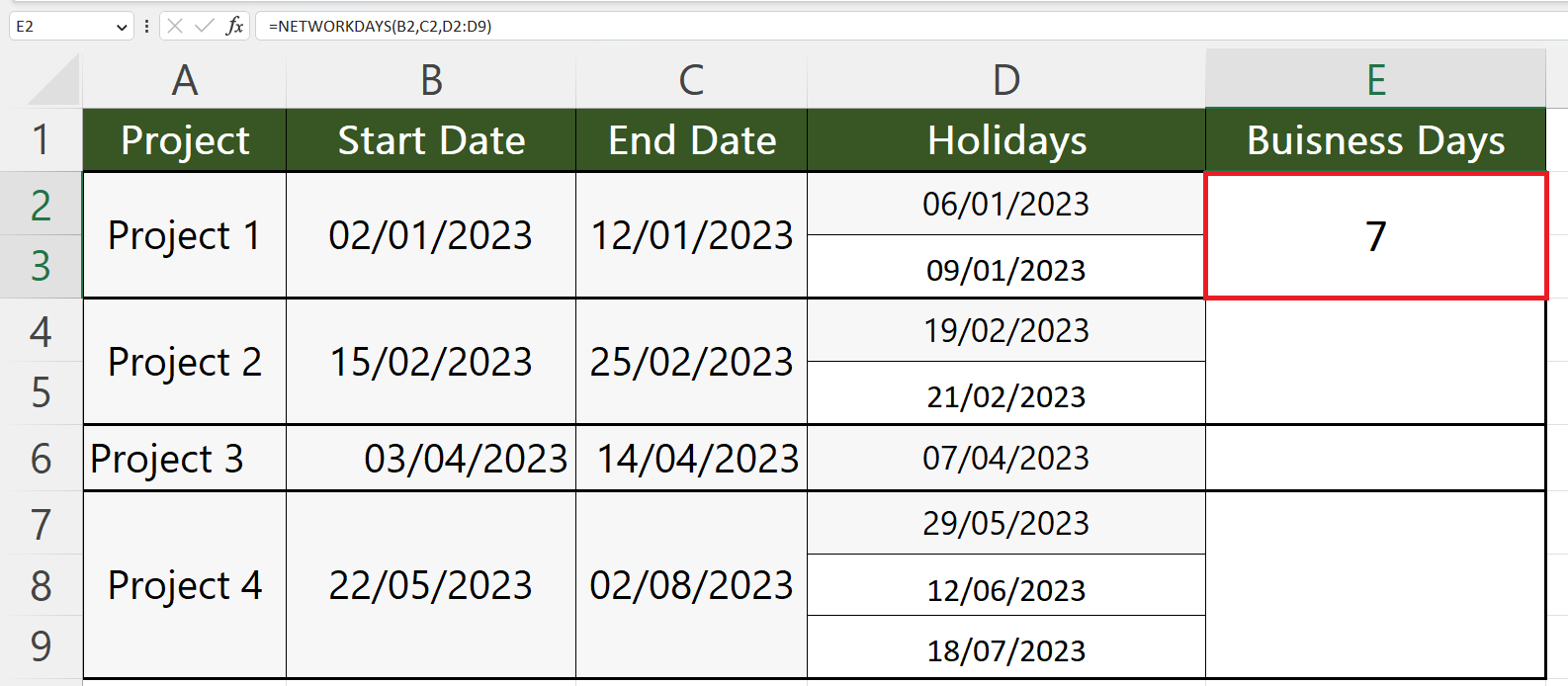# How to calculate business days in Microsoft Excel

In this tutorial, we will learn how to calculate business days in Microsoft Excel. In Microsoft Excel calculating business days is a very common task that can be performed by utilizing the built-in NETWORKDAYS function.

## Syntax of NETWORKDAYS

The syntax of NETWORKDAYS function explained below.

Let’s say we have a data set that represents starting and ending dates of some projects and the holidays that lie in between. We want to calculate the number of working days for each project.Calculating business days in Microsoft Excel refers to determining the number of working days between two given dates, excluding specified holidays. This calculation is commonly used in various scenarios, such as tracking project timelines, estimating delivery dates, or calculating durations excluding non-working days.

### Step 1 – Choose an Empty Cell– Choose an empty cell where you want to calculate the business days.

### Step 2 – Utilize the “NETWORKDAYS” Function– Utilize the NETWORKDAYS function in the cell.
– The structure of the function will be:
NETWORKDAYS(B2, C2, D2:D3)
– Where cell B2 holds the start date, cell C2 holds the end date of the project and the third parameter is the range containing the holidays.

### Step 3 – Strike the Enter Key– Strike the Enter key to calculate the business days.

### Step 4 – Utilize the Same Steps to Calculate the Business Days for Each Project–  Utilize the above-mentioned steps for each project to calculate the business days.